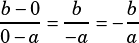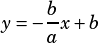Which of the following is an equation of the line with x-intercept a and y-intercept b, where ab is not equal to 0?
A) y= ax+b
B) y=bx +a
C) y= (a/b)x +a
D) y= (b/a)x +b
E) y= (-b/a)x =b

You have to reverse engineer this one a bit. You know, based on the question, that the points (a, 0) and (0, b) are on the graph. From that, you can find the slope:Armed with that slope, and with the y-intercept of b, you can write your slope-intercept equation:Note that this is also a question that’s quite susceptible to plugging in, if that helps you visualize it. Pick values for a and b, then plug those into each answer choice, graph them, and see which line actually goes through the points it’s supposed to. 🙂